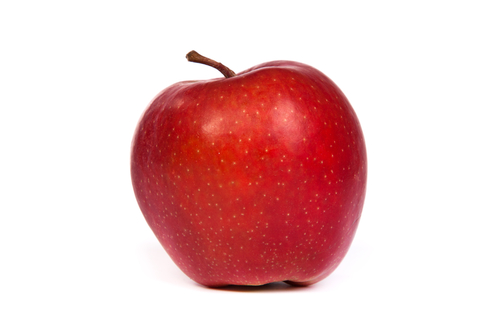#Teacher Note: Names for Formal and Actual Parameters¶

A formal parameter is the name of the input value to a procedure, while an actual parameter is the value itself that is passed to the procedure when it is called. In the following example, name is the formal parameter, while "John" is the actual parameter:

Activity: CodeLens 1 (ActualVsFormal1)

Of course, instead of passing the literal string "John", we can alternatively pass a variable name:

Activity: CodeLens 2 (ActualVsFormal2)

In this case, name is the formal parameter, while person is the actual parameter.

Depending on the examples of procedure definitions and calls the students encounter, some may think that it is important to the computer whether or not the names of the formal and actual parameters match.

Correct understanding In fact, it does not matter if the names do not match, as we see in the example above. Making use of our variables-as-boxes analogy, what is actually passed to the procedure is a copy of the value contained in the box, not the box itself. For both calls to greet above — greet("John") and greet(person) — the same value is passed: "John".

Run the above code in CodeLens to see that the value "John" is copied to the variable name from the variable person .

Conversely, the following example demonstrates that you can also use the same names for the formal and actual parameters:

Activity: CodeLens 3 (ActualVsFormal3)

This example might seem a bit more confusing, since we use name twice. We understand, however, that the name of the actual parameter does not matter, since it is a copy of the value that we are passing. This code, then, will behave exactly as the previous two examples.

How do we make sense then of name being used twice? If you run this example in CodeLens, you’ll see that what actually happens is that there are two name variables. When greet is called, the value of name in the Global frame is copied into the variable name inside the greet frame.

How to tell if a student has this misconception Show all three of the above examples, and ask the students if any of them would produce an error.